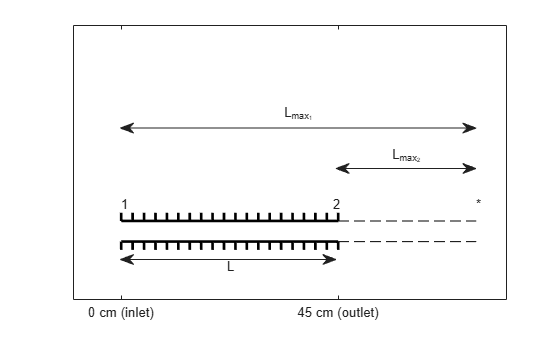# Analyzing Flow with Friction Through an Insulated Constant Area Duct

This example shows how to implement a steady, viscous flow through an insulated, constant-area duct using Aerospace Toolbox software. This flow is also called Fanno line flow.

### Problem Definition

This section describes the problem to be solved. It also provides necessary equations and known values.

Fanno line flow is the modeling of perfect gas flow through a constant-area duct that does not change with time and which is adiabatic. Wall friction is the main mechanism for the change of the flow variables. This example looks at Fanno flow of air entering a 3 centimeter diameter pipe that is 45 centimeters long at a Mach number of 0.6. The conditions at the inlet, also called station 1, are static pressure of 150 kilopascals and static temperature of 300 Kelvin. The duct is assumed to have a coefficient of friction of 0.02. Calculate the Mach number, static pressure, and static temperature at the exit of the duct or station 2.

`ductPicture = plotFannoFlowDuct;`The given information in the problem is:

```ductLength = 0.45; % Length of the duct [m] diameter = 0.03; % Diameter of the duct [m] inletMach = 0.6; % Mach number at the duct inlet [dimensionless] inletPressure = 150; % Static pressure at the duct inlet [kPa] inletTemperature = 300; % Static temperature at the duct input [K] frictionCoeff = 0.02; % Duct friction coefficient [dimensionless]```

The fluid is air which has the following specific heat ratio.

`k = 1.4; % Specific heat ratio [dimensionless]`

### Understanding the Fanno Parameter

The Fanno parameter is a dimensionless quantity that indicates how much influence friction will have while the fluid is flowing through the duct. For a given duct, the Fanno parameter is defined as

`$Fanno\phantom{\rule{0.2777777777777778em}{0ex}}parameter=\frac{fL}{{D}_{h}}$`

where

`$L=Length\phantom{\rule{0.2777777777777778em}{0ex}}of\phantom{\rule{0.2777777777777778em}{0ex}}the\phantom{\rule{0.2777777777777778em}{0ex}}duct$`

`${D}_{h}=\frac{4*Cross-sectional\phantom{\rule{0.2777777777777778em}{0ex}}area}{Perimeter\phantom{\rule{0.2777777777777778em}{0ex}}of\phantom{\rule{0.2777777777777778em}{0ex}}cross-section}=Hydraulic\phantom{\rule{0.2777777777777778em}{0ex}}diameter$`

For the circular pipe, assume the hydraulic diameter is the inner diameter of the pipe. The friction coefficient, f, is given by the following expression:

`$f=\frac{4{\tau }_{f}}{\frac{1}{2}\rho {V}^{2}}$`

where

`${\tau }_{f}=Shear\phantom{\rule{0.2777777777777778em}{0ex}}stress\phantom{\rule{0.2777777777777778em}{0ex}}due\phantom{\rule{0.2777777777777778em}{0ex}}to\phantom{\rule{0.2777777777777778em}{0ex}}wall\phantom{\rule{0.2777777777777778em}{0ex}}friction$`

`$\rho =Density$`

`$V=Velocity$`

Note, this example uses the convention in which a factor of four is not visible in the Fanno parameter. This convention defines the friction coefficient as four times the skin friction over the dynamic pressure. Friction will have a greater effect on the flow in a long duct than in a short duct because the flow is impeded by more wall surface friction. Additionally, friction is more dominant when the duct is narrow. This is because the boundary layer affects a larger portion of the flow along the walls than when the duct width is large.

Friction is an energy loss that generates entropy (irreversibility) in the system. The increase in entropy causes the flow to tend towards the choked condition (Mach = 1). The choked condition occurs if the length of the duct is long enough. For a given Mach number and specific heat ratio, the reference Fanno parameter for choked flow is

`$\frac{f{L}_{max}}{{D}_{h}}=\frac{1-{M}^{2}}{\gamma {M}^{2}}+\frac{\gamma +1}{2\gamma }\phantom{\rule{0.2222222222222222em}{0ex}}ln\left(\frac{{M}^{2}}{\frac{2}{\gamma +1}\left[1+\frac{\gamma -1}{2}{M}^{2}\right]}\right)$`

where

`$\gamma =k=Specific\phantom{\rule{0.2777777777777778em}{0ex}}heat\phantom{\rule{0.2777777777777778em}{0ex}}ratio$`

### Using the Fanno Parameter and flowfanno to Solve for the Flow Properties at the Inlet

This example provides the length of the duct, the diameter of the duct, and the friction coefficient. Therefore, the actual Fanno parameter of the duct can be computed as follows:

`fannoParameter = frictionCoeff * ductLength / diameter;`

This example also provides the Mach number and specific heat ratio. This enables you to calculate the reference Fanno parameter for the inlet condition, the inlet temperature ratio, and the inlet pressure ratio. Use the `flowfanno` function:

`[~, inletTempRatio, inletPresRatio, ~, ~, ~, inletFannoRef] = flowfanno(k, inletMach);`

`$inletTempRatio=\frac{{T}_{1}}{{T}_{1}^{*}}$`

`$inletPresRatio=\frac{{p}_{1}}{{p}_{1}^{*}}$`

`$inletFannoRef={\left(\phantom{\rule{-0.16666666666666666em}{0ex}}\phantom{\rule{-0.16666666666666666em}{0ex}}\frac{f{L}_{max}}{{D}_{h}}\phantom{\rule{-0.16666666666666666em}{0ex}}\phantom{\rule{-0.16666666666666666em}{0ex}}\right)}_{1}$`

where:

• The subscript indicates the flow station.

• Unstarred quantities are the local values of the given variables.

• Starred quantities are referenced value of the given variables if the flow is brought to the choked condition.

The length of the inlet reference Fanno parameter, also called inlet max length, is the length that the pipe needs to be to have choked flow for a given inlet condition. If the actual length is less than the inlet max length, an extension to the pipe is needed for choked flow. This choked flow corresponds to a reference Fanno parameter for the outlet. Since the diameter and friction coefficient are given in the problem statement, only the lengths vary in the following equation for the outlet reference Fanno parameter:

`${\left(\phantom{\rule{-0.16666666666666666em}{0ex}}\phantom{\rule{-0.16666666666666666em}{0ex}}\frac{f{L}_{max}}{{D}_{h}}\phantom{\rule{-0.16666666666666666em}{0ex}}\phantom{\rule{-0.16666666666666666em}{0ex}}\right)}_{2}={\left(\phantom{\rule{-0.16666666666666666em}{0ex}}\phantom{\rule{-0.16666666666666666em}{0ex}}\frac{f{L}_{max}}{{D}_{h}}\phantom{\rule{-0.16666666666666666em}{0ex}}\phantom{\rule{-0.16666666666666666em}{0ex}}\right)}_{1}-\frac{fL}{{D}_{h}}$`

`outletFannoRef = inletFannoRef - fannoParameter;`

### Calculating the Flow Properties at the Outlet with the flowfanno Function

Next, use `flowfanno` to calculate the flow ratios at the outlet station. The third input, fannosub, indicates that the second input, outletFannoRef, is a subsonic Fanno parameter input.

`[outletMach, outletTempRatio, outletPresRatio] = flowfanno(k, outletFannoRef, 'fannosub');`

`$outletTempRatio=\frac{{T}_{2}}{{T}_{2}^{*}}$`

`$outletPresRatio=\frac{{p}_{2}}{{p}_{2}^{*}}$`

Use the temperature ratios found at the inlet and outlet to calculate the temperature and pressure at the outlet. The reference conditions are the same at both stations because the duct is insulated. In addition, assume that the effects of friction act on both stations in the same manner. As a result, we have

`${T}_{1}^{*}={T}_{2}^{*}$`

`${p}_{1}^{*}={p}_{2}^{*}$`

Therefore, the temperature at the outlet and the pressure at the outlet are

`${T}_{2}={T}_{1}\frac{{T}_{1}^{*}}{{T}_{1}}\frac{{T}_{2}}{{T}_{2}^{*}}$`

`outletTemperature = inletTemperature / inletTempRatio * outletTempRatio;`

`${p}_{2}={p}_{1}\frac{{p}_{1}^{*}}{{p}_{1}}\frac{{p}_{2}}{{p}_{2}^{*}}$`

`outletPressure = inletPressure / inletPresRatio * outletPresRatio;`

The values that we want to calculate are

`outletMach % [dimensionless]`
```outletMach = 0.7093 ```
`outletTemperature % [K]`
```outletTemperature = 292.2018 ```
`outletPressure % [kPa]`
```outletPressure = 125.2332 ```

For Fanno line flow where the inlet flow is subsonic, the temperature and pressure always decrease through the duct. For all Fanno line flow cases, the Mach number moves closer to one.

### Reference

 James, J. E. A., "Gas Dynamics, Second Edition", Allyn and Bacon, Inc, Boston, 1984.